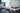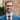# Prefer const over let

###### Cory Mortimer Martin | July 6, 2020
< Back to blog

When defining variables, it is best to use `const` wherever possible. The `const` keyword signals to the next developer that the variable will not be reassigned. This reduces the amount of context that the developer needs to keep track of.

Below is a contrived example only using the `let` keyword to declare and define variables:

``````let myFunction = () => {
let a = 1
let b = 2
let c = 3
let d = 4
let r

a = a + b + c + d

r = `The result is \${a}`

return r
}
``````

Here is the above example using `const` and `let` where appropriate:

``````const myFunction = () => {
let a = 1
const b = 2
const c = 3
const d = 4
let r

a = a + b + c + d

r = `The result is \${a}`

return r
}
``````

In the second example, the `const` keyword frees up mental capacity allowing developers to understand the code more quickly and to think about opportunities to improve the code.

...